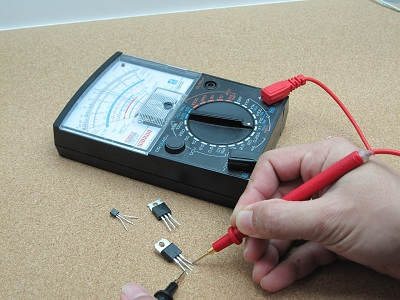Learning Objective

In this lesson we will learn how electricity can be measured in terms of voltage and current.

Learning Outcomes

By the end of this lesson you will be able to:

• Define voltage and explain how it can be measured using a voltmeter.

• Define current and explain how it can be measured using an ammeter.

• Compare the distribution of voltage and current in series and parallel circuits.

• Explain the use of transformers in electrical devices.

## Contents

1 | Measuring Electricity

2 | Voltage

3 | Current

4 | Electricity Supply in Australia

5 | Transformers

6 | Voltage and Current in Series and Parallel Circuits

7 | Comparing Parallel and Series Circuits with Multiple Light Bulbs

8 | Summary